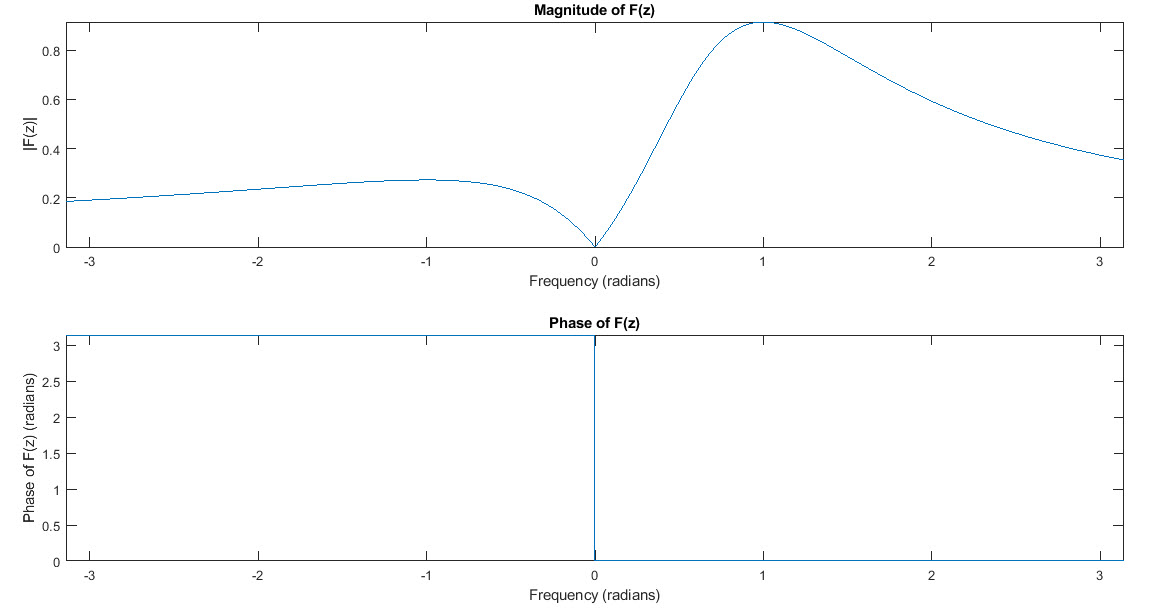# Computing the Z-transform in MATLAB

The Z-transform is an essential tool in digital signal processing (DSP) for analyzing and processing discrete-time signals. It is a mathematical transform that maps a discrete-time signal in the time domain to a complex-valued function in the frequency domain. In this blog post, we will explore how to use MATLAB to compute and plot the Z-transform of a discrete-time signal.

The Z-transform of a discrete-time signal x(n) is defined as the infinite sum of the signal values weighted by powers of a complex variable z.  $$X(z) = \sum_{n=-\infty}^{\infty}x(n) z^{-n}$$

## Computing the Z-transform in MATLAB

MATLAB provides a convenient way to compute the Z-transform of a discrete-time signal using the ztrans function. The syntax of the function is as follows:

F = ztrans(f, n, z)

where f is the symbolic expression of the discrete-time signal, n is the symbolic variable representing the time index, and z is the symbolic variable representing the complex frequency variable. The output F is the symbolic expression of the Z-transform of the signal.

For example, let's consider discrete sine signal, then the following code:

syms n z
f = sin(n);
F = ztrans(f, n, z);

gives F as,

$$F=\frac{z\,\mathrm{sin}\left(1\right)}{z^2 -2\,\mathrm{cos}\left(1\right)\,z+1}$$

Here, we define a symbolic variable n to represent the time index and a symbolic variable z to represent the complex frequency variable. We then define the signal f as sin(n). Finally, we use the ztrans function to compute the Z-transform of the signal and store it in the variable F.

## Plotting the Z-transform in MATLAB

Once we have computed the Z-transform of a signal, we can plot its magnitude and phase as a function of the normalized frequency using MATLAB's plotting functions. In this example, we will use the fplot function to plot the magnitude and phase of the Z-transform of the signal.

To plot the magnitude of the Z-transform, we can use the following code:

fplot(abs(F), [-pi, pi]);
title('Magnitude of F(z)');
ylabel('|F(z)|');

Here, we use the fplot function to plot the absolute value (abs) of the Z-transform F over the frequency range from -π to π. We then set the title and axis labels of the plot.

Similarly, to plot the phase of the Z-transform, we can use the following code:

fplot(angle(F), [-pi, pi]);
title('Phase of F(z)');
ylabel('Phase of F(z) (radians)');

Here, we use the fplot function to plot the phase (angle) of the Z-transform F over the same frequency range. We then set the title and axis labels of the plot.

Complete Matlab Z-Transform code

clear
syms n z
f = sin(n);
F = ztrans(f, n, z);
F

% Plot the magnitude of F(z)
fplot(abs(F), [-pi, pi]);
title('Magnitude of F(z)');
ylabel('|F(z)|');

% Plot the phase of F(z)
fplot(angle(F), [-pi, pi]);
title('Phase of F(z)');
ylabel('Phase of F(z) (radians)');
####Conclusion
In this blog post, we explored how to use MATLAB to compute and plot the Z-transform of a discrete-time signal. We learned how to use the ztrans function to compute the Z-transform and the fplot function to plot its magnitude and phase as functions of the normalized frequency. These tools are essential for analyzing and processing discrete-time signals in digital signal processing.